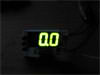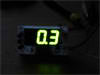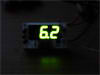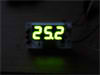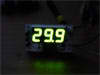# 3 Digits Digital Ammeter

Here is a digital Ammeter based on PIC16F684 and ACS712 current sensor. The measured DC or AC current will display on 3 digits 7-segment with resolution 100 mA. The current sensor in this project is ACS712ELCTR-30A-T from Allegro(I got it from Amazon). It can measure the AC or DC current up to 30A with 66 mV/A output sensitivity. This project is identical to my volt meter project except the microcontroller is not the same.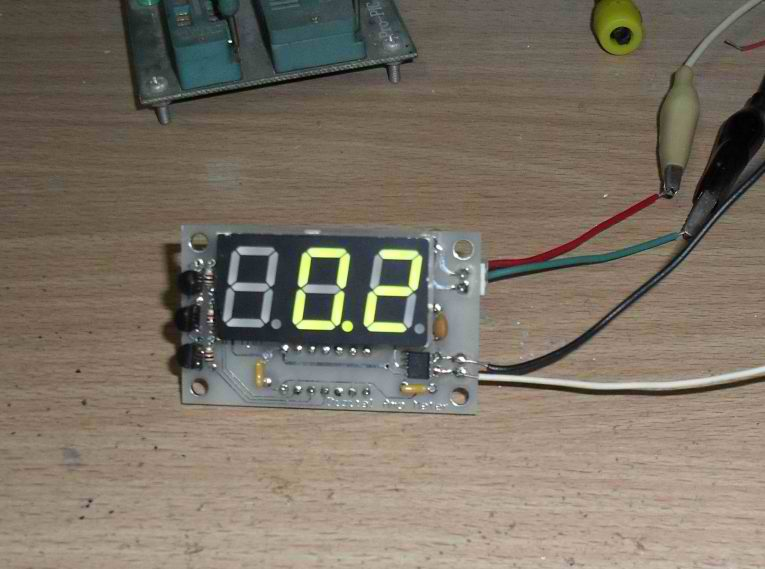Schematic

The microcontroller PIC16F684 reading analog value from the ACS712 current sensor output and convert to current for displaying on 7-segment. All 7-segments are common anode type and driven by PNP transistors. Actually, this is circuit suitable for measuring DC current e.g. solar panel to battery, battery to load.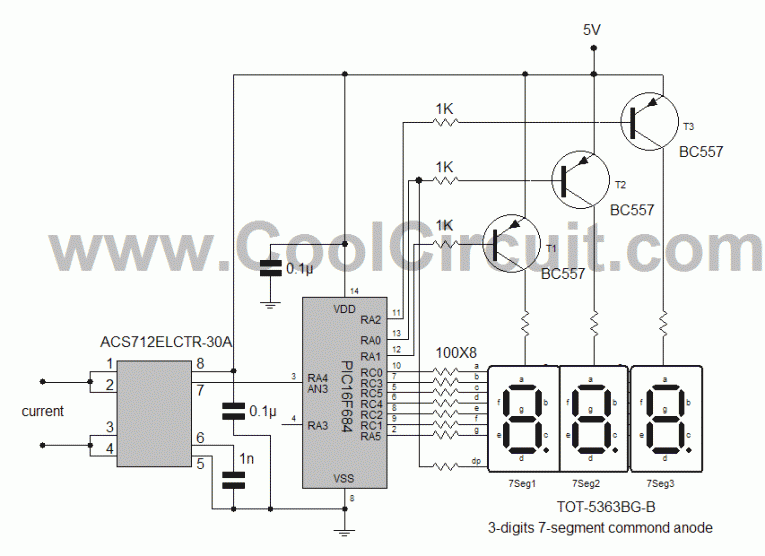Calculating volt to amp for Vcc 5V

From ACS712 data sheet we know :

0 A = Vcc/2 = 2.5V or 512 analog counts or 0x200.
Sensitivity 66mV/A for 30A version.

So
Volt at 30A = 2.5V + (66mV/A x 30A) = 4.48V or 917.504 analog counts
Volt at -30A = 2.5v – (66mV/A x 30A) = 0.52V or 106.5 analog counts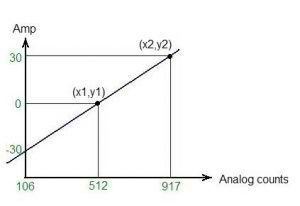With basic math linear equation:
y = mx + b
———– Equ. 1
m = (y2 – y1) / (x2-x1) ————-Equ.2

So we get
m = (30-0) / (917 – 512) = 0.074

substitute m in Equ. 1 at output 0A, we will get b

0 = 0.074*512 + b
b = -37.888

thus, the final equation use in software is :

A = 0.074*(analog_counts) – 37.888

Example :
if analog counts = 512 we get 0 A
if analog counts = 917 we get about +30A
if analog counts = 106 we get about -30A